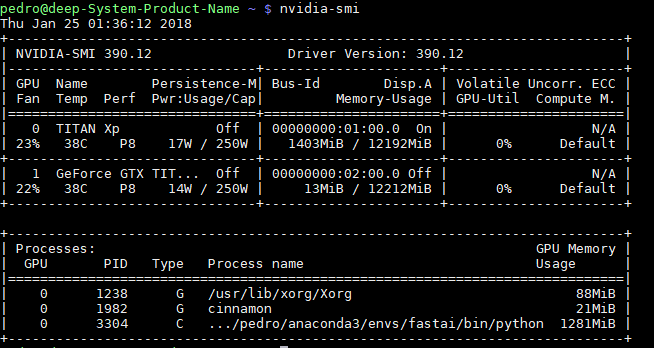# GPU memory not being freed after training is over

I was checking my GPU usage using nvidia-smi command and noticed that its memory is being used even after I finished the running all the code in lesson 1 as shown in the figure bellowThe memory is only freed once I restart the jupyter kernel.

Is this the usual behavior?

How can I free this memory without needing to restart the kernel?

Thanks

1 Like

Use gpustat -p to check process Id and memory used and then kill that process

It is not memory leak, in newest PyTorch, you can use torch.cuda.empty_cache() to clear the cached memory. - jdhao

11 Likes

After deleting some variables and using torch.cuda.empty_cache() I was able to free some memory but not all of it.
Here is a code example:

from fastai.imports import *
from fastai.transforms import *
from fastai.conv_learner import *
from fastai.model import *
from fastai.dataset import *
from fastai.sgdr import *
from fastai.plots import *
PATH = “data/dogscats/”

sz=224
arch=resnet34

data = ImageClassifierData.from_paths(PATH, tfms=tfms_from_model(arch, sz))
learn = ConvLearner.pretrained(arch, data, precompute=True)
learn.fit(0.01, 3)

% running nvidia-smi -> 689MB used

torch.cuda.empty_cache()

% running nvidia-smi -> 687MB used

del data, learn
torch.cuda.empty_cache()

% running nvidia-smi -> 571MB used

Any ideas what could be using the rest of the memory?

1 Like

Thanks for the answer, but killing the process is the same as closing the notebook. I was hoping to be able to free the memory without needing to kill the whole thing.

The consensus in that thread was that PyTorch will reuse some of the memory that nvidia-smi says is taken. I don’t know how to tell how much memory is actually taken. I’d only focus on this situation if I were getting out of memory errors.

Use this:

``````def pretty_size(size):
"""Pretty prints a torch.Size object"""
assert(isinstance(size, torch.Size))
return " × ".join(map(str, size))

def dump_tensors(gpu_only=True):
"""Prints a list of the Tensors being tracked by the garbage collector."""
import gc
total_size = 0
for obj in gc.get_objects():
try:
if torch.is_tensor(obj):
if not gpu_only or obj.is_cuda:
print("%s:%s%s %s" % (type(obj).__name__,
" GPU" if obj.is_cuda else "",
" pinned" if obj.is_pinned else "",
pretty_size(obj.size())))
total_size += obj.numel()
elif hasattr(obj, "data") and torch.is_tensor(obj.data):
if not gpu_only or obj.is_cuda:
print("%s → %s:%s%s%s%s %s" % (type(obj).__name__,
type(obj.data).__name__,
" GPU" if obj.is_cuda else "",
" pinned" if obj.data.is_pinned else "",
" volatile" if obj.volatile else "",
pretty_size(obj.data.size())))
total_size += obj.data.numel()
except Exception as e:
pass
print("Total size:", total_size)
``````

It shows the tensors that are still in use by your notebook. If this list is (mostly) empty, then you have freed all the memory you can free.

24 Likes

Ok. Seems reasonable enough

dump_tensors() outputs the total tensor size as being equal to 0

That’s a handy function - thanks!

1 Like

how do you avoid that a tensor sticks around in memory? I try do delete unused tensors on-the-go using del tensor within my forward() function. But somehow the function still shows that there are many tensors occupying the GPU. also, i have the problem that when using a multiprocessing dataloader, after keyboardinterrupting, the subprocesses still hang around and the gpu cache is permanently blocked until restarting the kernel. did not find a better way to do it so far than restarting. however, restarting is kind of annoying, because i need to reload my dataset every time from a mongo db, which takes dozens of minutes.

also, when using your function, i see sth like:

``````- parameter / gpu pinned / shape of tensor
- tensor / gpu pinned / shape of tensor
``````

Is it possible to print the names of the variables? So far, I can only infer from their shapes what statements they result from.

Thanks!